Courses

# Test: Deflection of Beams - 1

## 10 Questions MCQ Test Topicwise Question Bank for Civil Engineering | Test: Deflection of Beams - 1

Description
This mock test of Test: Deflection of Beams - 1 for Civil Engineering (CE) helps you for every Civil Engineering (CE) entrance exam. This contains 10 Multiple Choice Questions for Civil Engineering (CE) Test: Deflection of Beams - 1 (mcq) to study with solutions a complete question bank. The solved questions answers in this Test: Deflection of Beams - 1 quiz give you a good mix of easy questions and tough questions. Civil Engineering (CE) students definitely take this Test: Deflection of Beams - 1 exercise for a better result in the exam. You can find other Test: Deflection of Beams - 1 extra questions, long questions & short questions for Civil Engineering (CE) on EduRev as well by searching above.
QUESTION: 1

### A simply supported beam with width ‘b' and depth ‘d’ carries a central load W and undergoes deflection δ at the centre. If the width and depth are interchanged, the deflection at the centre of the beam would attain the value

Solution:

For a simply supported beam deflection, δ is given by,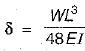∴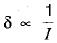Let δ1 be the deflection when the width and depth are interchanged,
∴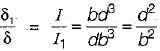∴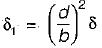QUESTION: 2

### Two identical cantileversare loaded as shown in figure. If slope at the free end of the cantilever in figure (a) is θ, the slope at the free end of the cantilever in figure (b) will be.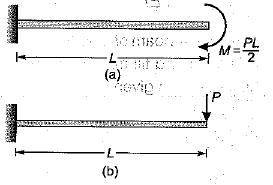Solution:

Slope in figure (a) is given by,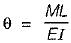Where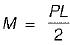∴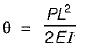Slope in figure (b) is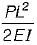∴ θb = θ

QUESTION: 3

### The given figure shows a cantilever of span L subjected to a concentrated load P and moment M at the free end. Deflection at the free end is given by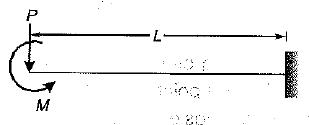Solution:

Deflection due to concentrated load acting at the end of cantilever= Pl3/3EI
Deflection due to moment acting at the end of cantilever= Ml2/2EI
Therefore, from principle of superposition, the deflection due to both moment and concentrated load acting at the end of cantilever will be Pl3/3EI + Ml2/2EI

QUESTION: 4

An overhang beam of uniform El is loaded as shown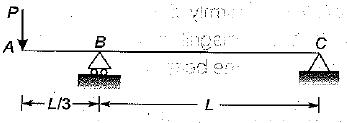The deflection at the free end will be

Solution:

The reaction RB at B is, ∑MC = 0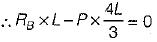⇒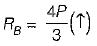∴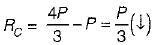∴ Total strain energy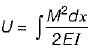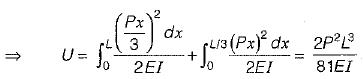So by Castigliano’s theorem, deflection at free end,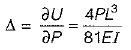QUESTION: 5

A horizontal beam of length l of uniform cross-section is pinned at ends A and B. At end A there is an anticlockwise moment M and at end B there is clockwise moment 2M. The slope at end A and end B are respectively.

Solution: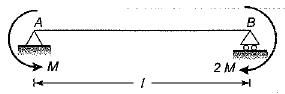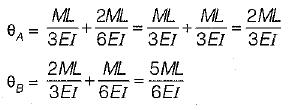QUESTION: 6

A cantilever beam of length ‘l’ carries a UDL ‘w’ from fixed end till mid span. The deflection at the free end is given by

Solution: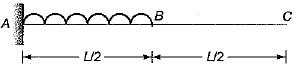The slope at mid span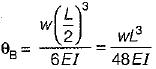∴ Deflection at free end,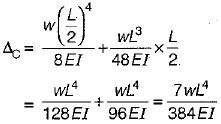QUESTION: 7

A cantilever beam of length ’L’ is subjected to a concentrated load P at free end what is the deflection at the centre of beam?

Solution: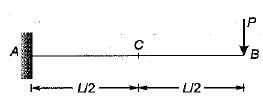Using Maxwell’s reciprocal theorem,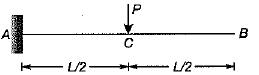Deflection at C when load is applied at B = Deflection at B when load is applied at C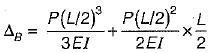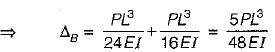QUESTION: 8

A 4-metre long beam, simply supported at its ends; carries a point load 'W at its centre. If the slope at the ends of beam is 1° then deflection at the centre of beam is

Solution:

Deflection at centre of beam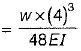Slope at ends of beam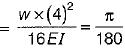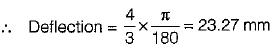QUESTION: 9

A simply supported beam AB of span ‘l’ has a uniform cross-section throughout. It carries a central concentrated load W and another load which is uniformly distributed over the entire span, its total magnitude being W. The maximum deflection in the beam is

Solution:

Maximum deflection will occur at centre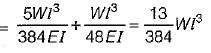QUESTION: 10

A cantilever beam of span ‘l’ and uniform flexural rigidity ‘EI’ is loaded with an upward force ‘W’ at the mid point and downward force ‘P’ at the free end. The deflection at the free end will be zero if

Solution: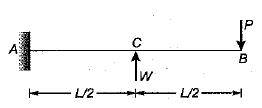Upward deflection at B due to load W,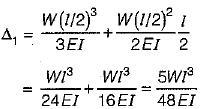Downward deflection at S due to load P,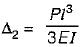For no deflection at B,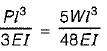⇒ W = 16P/5